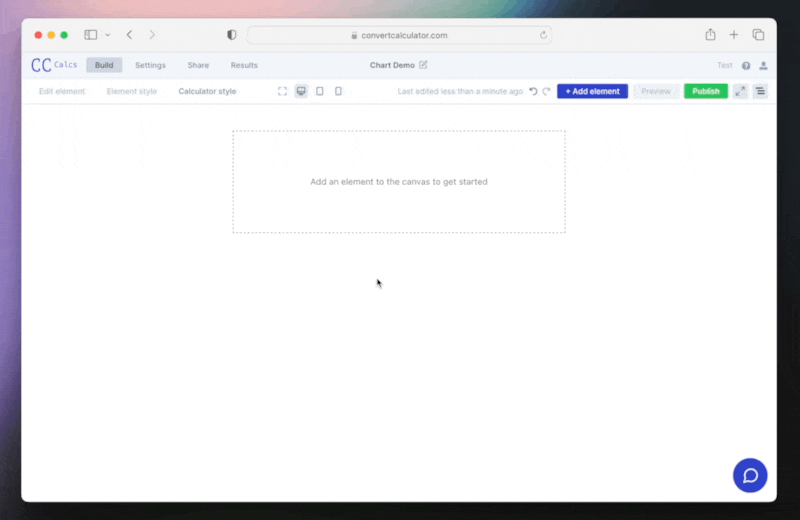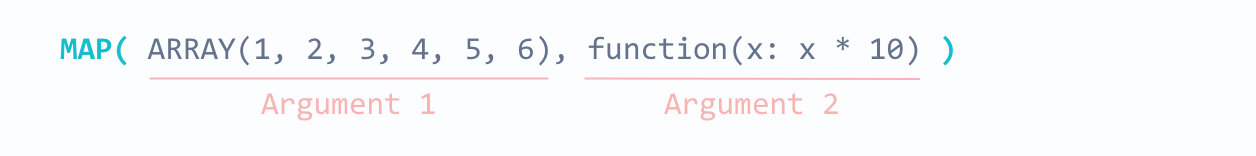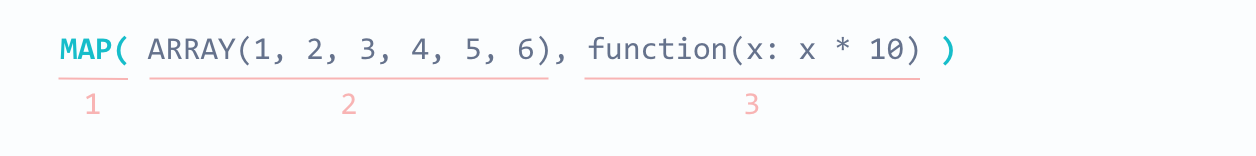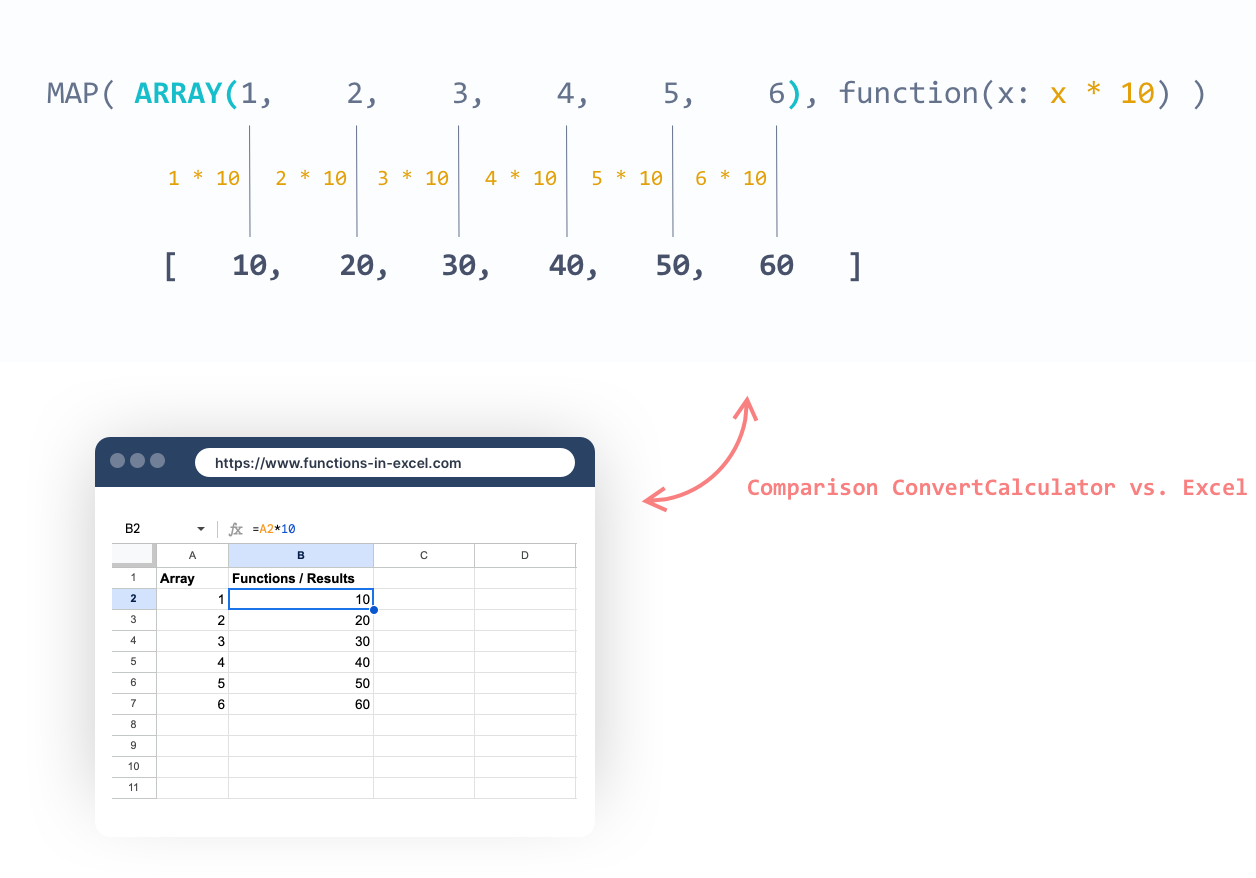# Charts

Use a chart to show and share your results more effectively.

Charts are great for presenting data and communicating information. They can be used to display values over time, compare categories, or show the composition of a whole. In ConvertCalculator, we have different types of charts available for different purposes. Most commonly line charts show trends over time, bar charts compare different categories, and pie charts show the composition of a whole.

You can add a chart element to your calculator by clicking “Add element” and selecting the “Chart” element under results.The chart already includes placeholder data for the horizontal axis labels and the dataset. Please clear this data before proceeding to build your chart.

## Chart types

We support the following chart types: Line, Bar, Stacked bar, Area, Donut, and Pie. To begin, choose the chart type that best suits your use case. You can switch between chart types at any time.

The labels for the vertical axis are set automatically. You can update the minimum and maximum y-axis scale, as well as the y-axis step size, in the 'Advanced chart settings' panel. Additionally, you can choose to hide the vertical labels from this panel as well.

The horizontal x-axis labels are optional. You can set them either via the panel by adding and naming labels in the table, or via a formula. Using a formula for your labels is an advanced option that provides more flexibility for setting the labels. You should choose this option when the number of data points depends on a configuration in your calculator.

To add a label formula, toggle the option ‘Use Formula for Labels’ and select the variable which contains the label formula. In case you do not yet have a variable containing your labels, you can add a new variable.

Labels formula example

See the example below for a formula you can use to create horizontal labels. For instance, if you're building a loan calculator and want the labels to display the separate years in the loan term, where the loan term is set by the question `QA`, use the following formula:

`FS`RANGE(1, QA)``

When the loan term is set to 10 years (`QA=10`), this function will output the following array/list: `(1, 2, 3, 4, 5, 6, 7, 8, 9, 10)`.

## Building a dynamic dataset

To create a chart in Excel or Google Sheets, you simply select a data range and then insert a chart via the menu. In ConvertCalculator, you can also use table data to create a chart. But there is an important difference. Cells in a spreadsheet can contain functions. These functions make spreadsheet cells dynamic. Cells in a ConvertCalculator table can only contain static data such as numbers, text, and email. The idea behind interactive content is that things on your screen change based on your choices.

To facilitate interactive charts in ConvertCalculator, we need a spreadsheet alternative to generate the dynamic chart input data.

With ConvertCalculator, you can create a dataset using custom and built-in functions. The formula outputs will provide you with a list of results. For example:

The combination of the following built-in and custom functions: `MAP( ARRAY(1, 2, 3, 4, 5, 6), function(x: x * 10) )` will give the output `[10, 20, 30, 40, 50, 60]`, which can be used as data for a chart. The function may seem difficult, but it's not too complex. The function consists of three parts:1. `MAP`: The MAP function repeats the custom function (3) for each value in the array (2). The output of the MAP function is a new array with the changed values.
2. `ARRAY`*: The array contains values that will be used in the custom function (3). The values in the array will replace `x` in the custom function.
3. `Custom function`: This simple function `x * 10` will be repeated for each value in the array (2) via the MAP function (1).

*To create a dynamic array (where the values update based on form inputs), use the `RANGE()` function. For example, `RANGE(1, QA)` creates an array that starts at 1 and adds a number to the array until `QA` is met. When the value of `QA` is `5`, the resulting array is `[1, 2, 3, 4, 5]`.

Let's break down the custom function to make it less intimidating:a. Function indicator: Use `function()` to indicate that you are writing a custom function in FormulaScript. b. Parameter: Substitute `x` with a value from the array. You can name the parameter `x` anything you like, such as `value`. c. Function: In this part, write the actual function where the argument `x` will be substituted. In this case, we chose a custom function, but you can also use one of our built-in functions like `ROUND(x, 5)`.

In summary:Here is how to build a dynamic dataset for your charts. For more information on building dynamic datasets, check out our Amortization Schedule Calculator. This template is quite advanced, as the calculations of the datasets overlap. You need results from one dataset to calculate the other.

## Static datasets

If your chart does not require values that will change, you can use static data inputs. To create an array, use the ARRAY function, like this: `ARRAY(10, 15, 40, 30, 50, 20)`. Alternatively, you can refer to a column in your datasheet (such as `DA_A`) or a table (such as `COLUMN(TABLE(TA), A)`).